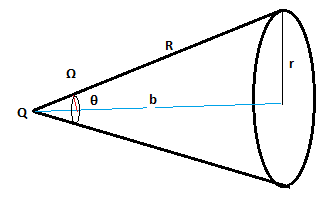# a point charge q is located on axis of disc of radius r at distance b from the plane of disc.if one-fourth of electric flux from charge passes through disc then.......(a)r = b    (b)r = 2bBadiuddin askIITians.ismu Expert
148 Points
13 years ago

Dear Dinesh

Draw a sphere whose center is at a distance b from the disk and which cover the diskwe know that flux throug a segment which makes an angel  θ at the center is

Φ=Q/2ε (1-cosθ)

where cosθ = b/(b2+r2)1/2

given flux passes in 1/4 th

so Q/2ε (1-cosθ)  =Q/4ε

1-cosθ = 1/2

cosθ =1/2

b/(b2+r2)1/2 =1/2

r =√3 b

Please feel free to post as many doubts on our discussion forum as you can.
If you find any question Difficult to understand - post it here and we will get you
the answer and detailed  solution very  quickly.

All the best.

Regards,

7 years ago
(it is disk not the spherical cap how can you take a sphere)

5 years ago
A 1000w laser beam is concentrated by a Lens of a cross-sectional area of 10^-5 cm^2 . find the corresponding amplitude of the electric fieldKushagra Madhukar
2 years ago
Hello studentAs we know total electric flux given by a point charge Q = Q/ε0
this flux is equally distributed in a solid angle of 4π

Now, if a disk makes an angle θ with the axis of charge, the solid angle covered by the disk
Ω = 2π(1- cosθ)

Hence electric flux through disk, ɸ =  Q/4πε0 x 2π(1- cosθ) = Q/2εx (1 – cosθ)
Given, this flux is ¼ of the flux given by charge Q
Hence,
Q/2εx (1 – cosθ) = Q/4ε0
or, cosθ = ½
or, θ = 60o
Hence, b/R = cos60o
or, b = R/2
or, R = 2b
or, b = (b2 + r2)1/2/2
or, 4b2 = b2 + r2
or, r2 = 3b2
or, r = √3 b

Hope it helps
Regards,
Kushagra